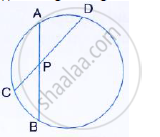Share

# I) in the Given Figure 3 × Cp = Pd = 9cm and Ap = 4.5 Cm Find Bp. - Mathematics

Course

#### Question

i) In the given figure 3 × CP = PD = 9cm and AP = 4.5 cm Find BP.#### Solution

i) Since two chords AB and CD intersect each other at P.
∴ AP ×  PB =  CP × PD
⇒ 4.5 ×  PB =  3×9 (3CP = 9cm ⇒ CP = 3cm)
⇒ PB = (3×9)/4.5= 6 cm

Is there an error in this question or solution?